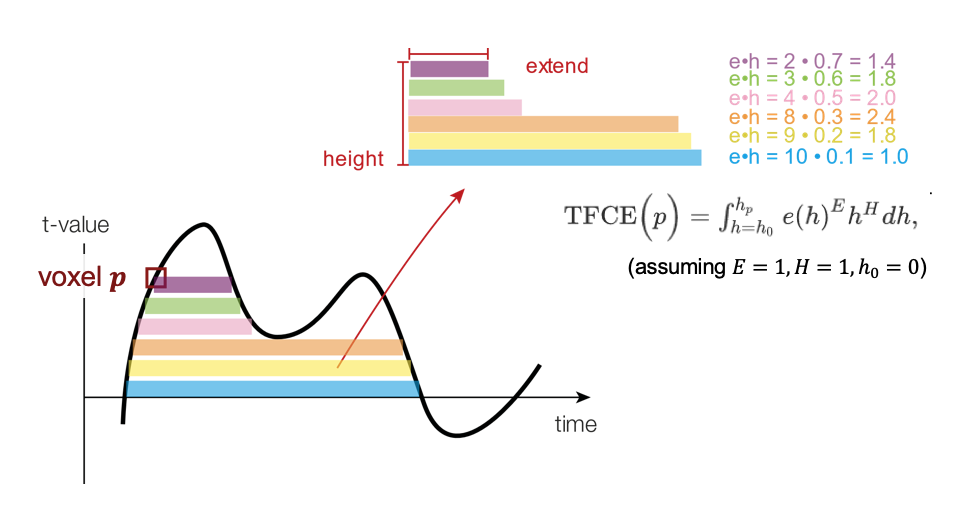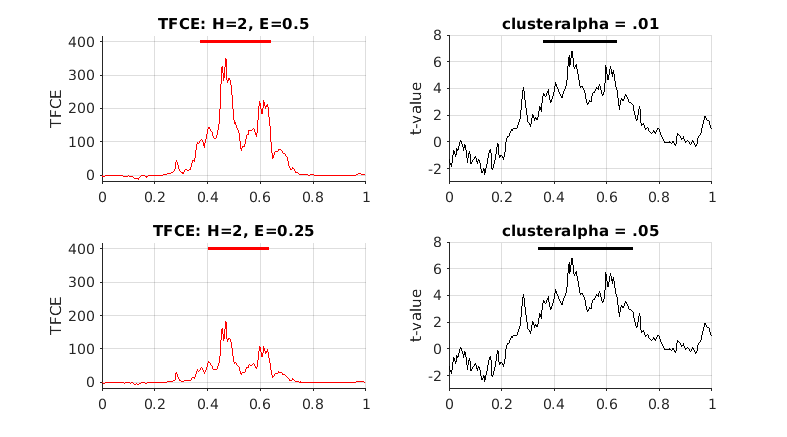Tags: example statistics

# Using threshold-free cluster enhancement for cluster statistics

This example explains how the threshold-free cluster enhancement (TFCE) method works for cluster statistics.

## Why is it useful?

When using clusting to correct for multiple comparisons, we need to define the cluster-forming threshold using the `cfg.clusterthreshold` and the `cfg.clusteralpha` options in ft_statistics_montecarlo. Different clusteralpha thresholds lead to clusters with a different spatial ot temporal extent, and thereby potentially to a different sensitivity of the subsequent permutation test. The threshold-free cluster enhancement method (TFCE) was introduced by Smith and Nichols (2009) to overcome this arbitrary threshold.

## How does it work?

Each voxel’s TFCE score is given by the sum of the scores of all “supporting sections” underneath it; as the height 𝒉 is incrementally raised from zero up to the height (signal intensity) 𝒉p of a given point 𝒑, the image is thresholded at 𝒉, and the single contiguous cluster containing p is used to define the score for that height 𝒉. This score is simply the height 𝒉 (raised to some power 𝑯, which is by default set to 2 in FieldTrip and can be adjusted via `cfg.tfce_H`) multiplied by the cluster extent 𝒆 (raised to some power 𝑬, which is by default set to 0.5 in FieldTrip and can be adjusted via `cfg.tfce_E`). For more detailed information, see Smith and Nichols (2009).TFCE schmeatic, adapted from Benedikt V. Ehinger (used under CC-BY)

## Example code

The following MATLAB code gives an example of the TFCE method. Moreover, we will compare the statistical output of the TFCE method with the conventional threshold based on `cfg.clusteralpha`.

The data used in this example is available from our download server.

``````load ERF_orig

Nsubj       = 10;
design      = zeros(2, Nsubj*2);
design(1,:) = [1:Nsubj 1:Nsubj];                % this is the uvar (unit-of-observation variable)
design(2,:) = [ones(1,Nsubj) ones(1,Nsubj)*2];  % this is the ivar (independent variable)

% channel neighbours are not really needed for the subsequent example, since we restrict the test to a single channel
% nevertheless, we will determine the channel neighbours here anyway
cfg          = [];
cfg.method   = 'template';
cfg.template = 'CTF151_neighb.mat';
nb           = ft_prepare_neighbours( cfg, allsubjFIC{1} );

%% Conventional cluster-based permutation test
cfg                  = [];
cfg.design           = design;
cfg.uvar             = 1;
cfg.ivar             = 2;
cfg.channel          = {'MLT12'};
cfg.latency          = [0 1];
cfg.method           = 'montecarlo';
cfg.statistic        = 'depsamplesT';
cfg.numrandomization = 'all';   % there are 10 subjects, so 2^10=1024 possible raondomizations
cfg.tail             = 0;
cfg.alpha            = 0.025;   % since we are testing two tails
cfg.neighbours       = nb;      % this will not be used, since we selected only a single channel
cfg.correctm         = 'cluster';
cfg.clusterstatistic = 'maxsum';
cfg.clusterthreshold = 'nonparametric_individual';
cfg.clustertail      = 0;
cfg.clusteralpha     = 0.01;
stat01 = ft_timelockstatistics(cfg, allsubjFIC{:}, allsubjFC{:});

cfg.clusteralpha     = 0.05;
stat05 = ft_timelockstatistics(cfg, allsubjFIC{:}, allsubjFC{:});

%% Cluster-based permutation test with TFCE method
cfg                  = [];
cfg.design           = design;
cfg.uvar             = 1;
cfg.ivar             = 2;
cfg.channel          = {'MLT12'};
cfg.latency          = [0 1];
cfg.method           = 'montecarlo';
cfg.statistic        = 'depsamplesT';
cfg.numrandomization = 'all';   % there are 10 subjects, so 2^10=1024 possible raondomizations
cfg.tail             = 0;
cfg.alpha            = 0.025;   % since we are testing two tails
cfg.neighbours       = nb;      % this will not be used, since we selected only a single channel
cfg.correctm         = 'tfce';
cfg.tfce_H           = 2;       % default setting
cfg.tfce_E           = 0.5;     % default setting
statA = ft_timelockstatistics(cfg, allsubjFIC{:}, allsubjFC{:});

cfg.tfce_H           = 2;
cfg.tfce_E           = 0.25;
statB = ft_timelockstatistics(cfg, allsubjFIC{:}, allsubjFC{:});
``````

Note that the TFCE method takes longer to compute than the conventional method. We can visualize and compare the slightly different statistical outputs given by these methods.

``````%% Visualize the results
figure(1), clf, hold on,
set(gcf, 'units','centimeters','position',[0 0 18 10] );
subplot(2,2,1), hold on, grid on,
title( 'TFCE: H=2, E=0.5' );
plot( statA.time, statA.stat_tfce, 'r');
ylabel( 'TFCE' );
ylim( [-20, 420] );
xticks( 0:0.2:1 );
set(gca, 'TickDir','out' );

subplot(2,2,3), hold on, grid on,
title( 'TFCE: H=2, E=0.25' );
plot( statB.time, statB.stat_tfce, 'r');
ylabel( 'TFCE' );
ylim( [-20, 420] );
xticks( 0:0.2:1 );
set(gca, 'TickDir','out' );

subplot(2,2,2), hold on, grid on,
title( 'clusteralpha = .01' );
plot( stat01.time, stat01.stat, 'k');
ylabel( 't-value' );
ylim( [-3, 8] );
xticks( 0:0.2:1 );
set(gca, 'TickDir','out' );

subplot(2,2,4), hold on, grid on,
title( 'clusteralpha = .05' );
plot( stat05.time, stat05.stat, 'k');
ylabel( 't-value' );
ylim( [-3, 8] );
xticks( 0:0.2:1 );
set(gca, 'TickDir','out' );
``````

## Output of the example code

When you inspect `stat01`, `stat05`, `statA` and `statB`, you will see that in this case the null-hypothesis of exchangeability of the data over the two conditions is rejected in all four.

Here is the expected output of the example code.